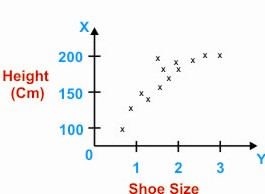HOME MATH DICTIONARY DOWNLOAD FEEDBACK DISCLAIMER
 Question: What is Scatter Diagram ? Answer: A scatter diagram is a diagram used in statistics to compare two quantities, often to show the correlation between them. For example a group of people each have their height and shoe size recorded as a point on a graph. Because these measurements have a high correlation the points are all close to a line. A scatterplot is a graphical summary of bivariate data (two variables X and Y), usually drawn before working out a linear correlation coefficient or fitting a regression line. A scatterplot will show up a linear or non-linear relationship between the two variables and whether or not there exist any outliers in the data.